# 5. Digital Image Processing Fundamentals

There’s more to it than meets the eye.
19th century proverb

Digital image processing is electronic data processing on a 2-D array of numbers. The array is a numeric representation of an image. A real image is formed on a sensor when an energy emission strikes the sensor with sufficient intensity to create a sensor output. The energy emission can have numerous possible sources (e.g., acoustic, optic, etc.). When the energy emission is in the form of electromagnetic radiation within the band limits of the human eye, it is called visible light [Banerjee]. Some objects will reflect only electromagnetic radiation. Others produce their own, using a phenomenon called radiancy. Radiancy occurs in an object that has been heated sufficiently to cause it to glow visibly [Resnick]. Visible light images are a special case, yet they appear with great frequency in the image processing literature.
Another source of images includes the synthetic images of computer graphics. These images can provide controls on the illumination and material properties that are generally unavailable in the real image domain.
This chapter reviews some of the basic ideas in digital signal processing. The review includes a summary of some mathematical results that will be of use in Chapter 15. The math review is included here in order to strengthen the discourse on sampling.

## 5.1. The Human Visual System

A typical human visual system consists of stereo electromagnetic transducers (two eyes) connected to a large number of neurons (the brain). The neurons process the input, using poorly understood emergent properties (the mind). Our discussion will follow the eye, brain and mind ordering, taking views with a selective focus.
The ability of the human eye to perceive the spectral content of light is called color vision. A typical human eye has a spectral response that varies as a function of age and the individual. Using clinical research, the CIE (Commission Internationale de L’Eclairage) created a statistical profile of human vision called the standard observer. The response curves of the standard observer indicate that humans can see light whose wavelengths have the color names red, green and blue. When discussing wavelengths for visible light, we typically give the measurements in nanometers. A nanometer is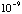meters and is abbreviated nm. The wavelength for the red, green and blue peaks are about 570-645 nm, 526-535 nm, and 444-445 nm. The visible wavelength range (called the mesopic range) is 380 to about 700-770 nm [Netravali] [Cohen].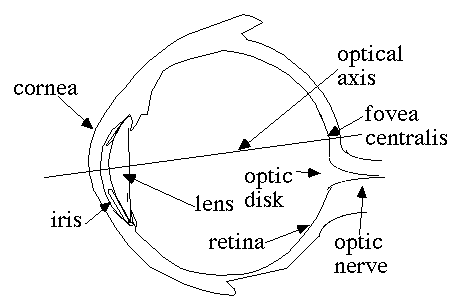Fig. 5-1. Sketch of a Human Eye
Fig. 5-1 shows a sketch of a human eye. When dimensions are given, they refer to the typical adult human eye unless otherwise stated. Light passes through the cornea and is focused on the retina by the lens. Physiological theories use biological components to explain behaviour. The optical elements in the eye (cornea, lens and retina) form the primary biological components of a photo sensor. Muscles are used to alter the thickness of the lens and the diameter of the hole covering the lens, called the iris. The iris diameter typically varies from 2 to 8 mm. Light passing through the lens is focused upon the retina. The retina contains two types of photo sensor cells: rods and cones.
There are 75 to 150 million rod cells in the retina. The rods contain a blue-green absorbing pigment called rhodopsin. Rods are used primarily for night vision (also called the scotopic range) and typically have no role in color vision [Gonzalez and Woods].
Cones are used for daylight vision (called the photopic range). The tristimulus theory of color perception is based upon the existence of three types of cones: red, green and blue. The pigment in the cones is unknown [Hunt]. We do know that the phenomenon called adaptation (a process that permits eyes to alter their sensitivity) occurs because of a change in the pigments in the cones [Netravali]. The retina cells may also inhibit each another from creating a high-pass filter for image sharpening. This phenomenon is known as lateral inhibition [Mylers].
The current model for the retinal cells shows a cone cell density that ranges from 900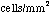to 160,000[Gibson]. There are 6 to 7 million cone cells, with the density increasing near the fovea. Further biological examination indicates that the cells are imposed upon a noisy hexagonal array [Wehmeier].
Lest one be tempted to count the number of cells in the eye and draw a direct comparison to modern camera equipment, keep in mind that even the fixated eye is constantly moving. One study showed that the eyes perform over 3 fixations per second during a search of a complex scene [Williams]. Further more, there is nearly a 180-degree field of view (given two eyes). Finally, the eye-brain interface enables an integration between the sensors’ polar coordinate scans, focus, iris adjustments and the interpretation engine. These interactions are not typical of most artificial image processing systems [Gonzalez and Woods]. Only recently have modern camcorders taken on the role of integrating the focus and exposure adjustment with the sensor.
The optic nerve has approximately 250,000 neurons connecting to the brain. The brain has two components associated with low-level vision operations: the lateral geniculate nucleus and the visual cortex. The cells are modeled using a circuit that has an inhibit input, capacitive-type electrical storage and voltage leaks, all driving a comparitor with a variable voltage output.
The capacitive storage elements are held accountable for the critical fusion frequency response of the eye. The critical fusion frequency is the rate of display whereby individual updates appear as if they are continuous. This frequency ranges from 10-70 Hz depending on the color [Teevan] [Netravali]. At 70 Hz, the 250,000-element optic nerve should carry 17.5 million neural impulses per second. Given the signal-to-noise ratio of a human auditory response system (80 dB), we can estimate that there are 12.8 bits per nerve leading to the brain [Shamma]. This gives a bit rate of about 224 Mbps. The data has been pre-processed by the eye before it reaches the optic nerve. This preprocessing includes lateral inhibition between the retinal neurons. Also, we have assumed that there is additive white Gaussian noise on the channel, but this assumption may be justified.
Physiological study has shown that the response of the cones is given by a Gaussian sensitivity for the cone center and surrounding fields. The overall sensitivity is found by subtracting the surrounding response from the center response. This gives rise to a difference of Gaussian expression which is discussed in Chap. 10. Further, the exponential response curve of the eye is the primary reason why exponential histogram equalization was used in Chap. 4.

## 5.2. Overview of Image Processing

An image processing system consists of a source of image data, a processing element and a destination for the processed results. The source of image data may be a camera, a scanner, a mathematical equation, statistical data, the Web, a SONAR system, etc. In short, anything able to generate or acquire data that has a two-dimensional structure is considered to be a valid source of image data. Furthermore, the data may change as a function of time.
The processing element is a computer. The computer may be implemented in a number of different ways. For example, the brain may be said to be a kind of biological computer that is able to perform image processing (and do so quite well!). The brain consumes about two teaspoons of sugar and 20 watts of power per hour. An optical element can be used to perform computation and does so at the speed of light (and with very little power). This is a fascinating topic of current research [Fietelson]. In fact, the injection of optical computing elements can directly produce information about the range of objects in a scene [DeWitt and Lyon].
Such computing elements are beyond the scope of this book. The only type of computer that we will discuss in this book is the digital computer. However, it is interesting to combine hybrid optical and digital computing. Such an area of endeavor lies in the field of photonics.
The output of the processing may be a display, created for the human visual system. Output can also be to any stream. In Java, a stream is defined as an uninterpreted sequence of bytes. Thus, the output may not be image data at all. For example, the output can be a histogram, a global average, etc. As the output of the program renders a higher level of interpretation, we cross the fuzzy line from image processing into the field of vision. As an example, consider that image processing is used to edge detect the image of coins on a table. Computer vision is used to tell how much money is there. Thus, computer vision will often make use of image processing as a sub-task.

### 5.2.1. Digitizing a Signal

Digitizing is a process that acquires quantized samples of continuous signals. The signals represent an encoding of some data. For example, a microphone is a pressure transducer that produces an electrical signal. The electrical signal represents acoustic pressure waves (sound).
The term analog refers to a signal that has a continuously varying pattern of intensity. The term digital means that the data takes on discrete values. Let s(t) be a continuous signal. Then, by definition of continuous,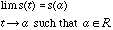(5.1)
We use the symbol R to denote the set of real numbers. Thus, which says that R is the set of all x such that x is a real number. We read (5.1) saying, in the limit, as t approaches a, such that a is a member of the set of real numbers,. The expression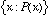is read as “the set of all x’s such that P(x) is true” [ Moore 64].
This is an iff (i.e., if and only if) condition. Thus, the converse must also be true. That is,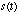is not continuous iff there exists a value, a such that:(5.2)
is true.
For example, ifhas multiple values at a, then the limit does not exist at a.
The analog-to-digital conversion consists of a sampler and a quantizer. The quantization is typically performed by dividing the signal into several uniform steps. This has the effect of introducing quantization noise . Quantization noise is given, in dB, using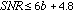(5.3)
where SNR is the signal-to-noise ratio and b is the number of bits. To prove (5.3), we follow [ Moore] and assume that the input signal ranges from -1 to 1 volts. That is,(5.3a)
Note that the number of quantization intervals is. The least significant bit has a quantization size of. Following [ Mitra], we obtain the bound on the size of the error with: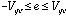(5.3b)
The variance of a random variable, X, is found bywhereis a probability distribution function. For the signal whose average is zero, the variance of (5.3b) is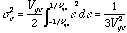(5.3c).
The signal-to-noise ratio for the quantization power is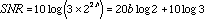(5.3d)
Hence the range on the upper bound for the signal-to-quantization noise power is(5.3).
Q.E.D.
In the above proof we assumed that uniform steps were used over a signal whose average value is zero. In fact, a digitizer does not have to requantize an image so that steps are uniform. An in-depth examination of the effects of non-linear quantization on SNR is given in [Gersho]. Following Gersho, we generalize the result of (5.3), defining the SNR as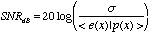(5.3e)
where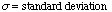andis the mean-square distortion defined by the inner product between the square of the quantization error for value x and the probability of value x. The inner product between e and p is given by(5.3f).
where(5.3g).
The inner product is an important tool in transform theory. We will expand our discussion of the inner product when we touch upon the topic of sampling.
We define Q(x) as the quantized value for x. Maximizing SNR requires that we select the quantizer to minimize (5.3f), given a priori knowledge of the PDF (if the PDF is available). Recall that for an image, we compute the PMF (using the Histogram class) as well as the CMF. As we shall see later, (5.3f) is minimized for k-level thresholding (an intensity reduction to k colors) when the regions of the CMF are divided into k sections. The color is then remapped into the center of each of the CMF regions. Hence (5.3f) provides a mathematical basis for reducing the number of colors in an image provided that the PDF is of zero mean (i.e, no DC offset) and has even symmetry about zero. That is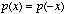. Also, we assume that the quantizer has odd symmetry about zero, i.e.,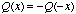.
A simple zero-memory 4-point quantizer inputs 4 decision levels and outputs 4 corresponding values for input values that range within the 4 decision levels. When the decision levels are placed into an array of double precision numbers, in Java (for the 256 gray-scale values) we write:
public void thresh4(double d[]) {
short lut[] = new short;
if (d ==0)
for ( int i=0; i < lut.length; i++) {
if (i < d) lut[i] = 0;
else if (i < d) lut[i] = ( short)d;
else if (i < d) lut[i] = ( short)d;
else if (i < d) lut[i] = ( short)d;
else lut[i] = 255;
System.out.println(lut[i]);
}
applyLut(lut);
}
We shall revisit quantization in Section 5.2.2.
Using the Java AWT’s Image class, we have seen that 32 bits are used, per pixel (red, green, blue and alpha). There are only 24 bits used per color, however. Section 5.2.2 shows how this relates to the software of this book.
Recall also that the digitization process led to sampling an analog signal. Sampling a signal alters the harmonic content (also known as the spectra) of the signal. Sampling a continuous signal may be performed with a pre-filter and a switch. Fig. 5-2 shows a continuous function,, being sampled at a frequency of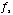.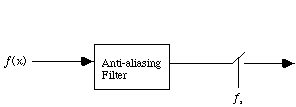Fig. 5-2. Sampling System
The switch in Fig. 5-2 is like a binary amplifier that is being turned on and off everyseconds. It multipliesby an amplification factor of zero or one. Mathematically, sampling is expressed as a pulse train,, multiplied by the input signal, i.e., sampling is..
To discuss the pulse train mathematically, we must introduce the notation for an impulse. The unit impulse, or Dirac delta, is a generalized function that is defined by(5.4)
where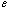is arbitrarily small. The Dirac delta has unit area about a small neighborhood located at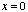. Multiply the Dirac delta by a function and it will sift out the values where the Dirac delta is equal to zero: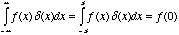(5.5)
This is called the sifting property of the Dirac delta. In fact, the Dirac delta is equal to zero whenever its argument is non-zero. To make the Dirac activate, given a non-zero argument, we bias the argument with an offset,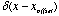. A pulse train is created by adding an infinite number of Dirac deltas together:(5.6)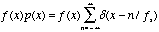(5.7)
To find the spectra of (5.7) requires that we perform a Fourier transform . The Fourier transform, just like any transform, performs a correlation between a function and a kernel. The kernel of a transform typically consists of an orthogonal basis about which the reconstruction of a waveform may occur. Two functions are orthogonal if their inner product=0. Recall that the inner product is given by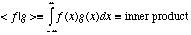(5.7a)
From linear algebra, we recall that a collection of linearly independent functions forms a basis if every value in the set of all possible values may be expressed as a linear combination of the basis set. Functions are linearly independent iff the sum of the functions is non-zero (for non-zero co-efficients). Conversely, functions are linearly dependent iff there exists a combination of non-zero coefficients for which the summation is zero. For example: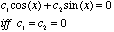(5.7b)
The ability to sum a series of sine and cosine functions together to create an arbitrary function is known an the super position principle and applies only to periodic waveforms. This was discovered in the 1800’s by Jean Baptiste Joseph de Fourier [Halliday] and is expressed as a summation of sine and cosines, with constants that are called Fourier coefficients.(5.7c)
We note that (5.7c) shows that the periodic signal has discrete spectral components. We find the Fourier coefficients by taking the inner product of the function, f(x) with the basis functions, sine and cosine. That is: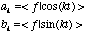(5.7d)
For an elementary introduction to linear algebra, see [Anton]. For a concise summary see [Stollnitz]. For an alternative derivation see [Lyon and Rao].
It is also possible to approximate an aperiodic waveform. This is done with the Fourier transform . The Fourier transform uses sine and cosine as the basis functions to form the inner product, as seen in (5.7a):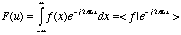(5.8).
By Euler’s identity,(5.9)
we see that the sine and cosine basis functions are separated by being placed on the real and imaginary axis.
Substituting (5.7) into (5.8) yields(5.10)
where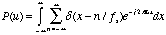(5.11)
The term(5.12)
defines a convolution. We can write (5.10) because multiplication in the time domain is equivalent to convolution in the frequency domain. This is known as the convolution theorem. Taking the Fourier transform of the convolution between two functions in the time domain results in(5.13)
which is expanded by (5.8) to yield:(5.13a)
Changing the order of integration in (5.13a) yields(5.13b)
with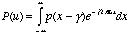(5.13c)
and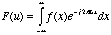(5.13d)
we get(5.14).
This shows that convolution in the time domain is multiplication in the frequency domain. We can also show that convolution in the frequency domain is equal to multiplication in the time domain. See [Carlson] for an alternative proof.
As a result of the convolution theorem, the Fourier transform of an impulse train is also an impulse train,(5.15)
Finally, we see that sampling a signal at a rate ofcauses the spectrum to be reproduced atintervals:(5.16)
(5.16) demonstrates the reason why a band limiting filter is needed before the switching function of Fig. 5-2. This leads directly to the sampling theorem which states that a band limited signal may be reconstructed without error if the sample rate is twice the bandwidth. Such a sample rate is called the Nyquist rate and is given by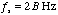.

### 5.2.2. Image Digitization

Typically, a camera is used to digitize an image. The modern CCD cameras have a photo diode arranged in a rectangular array. Flat-bed scanners use a movable platen and a linear array of photo diodes to perform the two-dimensional digitization.
Older tube type cameras used a wide variety of materials on a photosensitive surface. The materials vary in sensitivity and output. See [Galbiati] for a more detailed description on tube cameras.
The key point about digitizing an image in two dimensions is that we are able to detect both the power of the incident energy as well as the direction.
The process of digitizing an image is described by the amount of spatial resolution and the signal -to-noise ratio (i.e., number of bits per pixel) that the digitizer has. Often the number of bits per pixel is limited by performing a thresholding. Thresholding (a topic treated more thoroughly in Chap. 10) reduces the number of color values available in an image. This simulates the effect of having fewer bits per pixel available for display. There are several techniques available for thresholding. For the grayscale image, one may use the cumulative mass function for the probability of a gray value to create a new look-up table. Another approach is simply to divide the look-up table into uniform sections. Fig. 5-2 shows the mandrill before and after thresholding operation. The decision about when to increment the color value was made based on the CMF of the image. The number of bits per pixel (bpp), shown in Fig. 5-2, ranging from left to right, top to bottom, are: 1 bpp, 2 bpp, 3 bpp and 8 bpp. Keep in mind that at a bit rate of 28 kbps (the rate of a modest Internet connection over a phone line) the 8 bpp image (128x128) will take 4 seconds to download. Compare this to the uncompressed 1 bpp image which will take 0.5 seconds to download. Also note that the signal-to-noise ratio for these images ranges from 10 dB to 52 dB.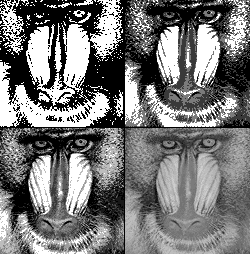Fig. 5-3. Quantizing with Fewer Bits Per Pixel
The code snippet allows the cumulative mass function of the image to bias decisions about when to increment the color value. The input to the code is the number of gray values, k. There are several methods to perform the quantization. The one shown in Fig. 5-3 is useful in edge detection (a topic covered in Chap. 10). The kgreyThresh method follows:
public void kgreyThresh(double k) {
Histogram rh = new Histogram(r,"red");
double cmf[] = rh.getCMF();
TransformTable tt = new TransformTable(cmf.length);
short lut[] = tt.getLut();
int q=1;
short v=0;
short dv = ( short)(255/k);
for ( int i=0; i < lut.length; i++) {
if (cmf[i] > q/k) {
v += dv;
q++; //(k == q+1)||
if (q==k) v=255;
}
lut[i]=v;
}
tt.setLut(lut);
tt.clip();
tt.print();
applyLut(lut);
}

### 5.2.3. Image Display

One display device that has come into common use is the cathode-ray tube (CRT). The cathode ray tube displays an image using three additive colors: red, green and blue. These colors are emitted using phosphors that are stimulated with a flow of electrons. Different phosphors have different colors (spectral radiance).
There are three kinds of television systems in the world today, NTSC, PAL and SECAM. NTSC which stands for National Television Subcommittee, is used in North America and Japan. PAL stands for phase alternating line and is used in parts of Europe, Asia, South America and Africa. SECAM stands for Sequential Couleur à Mémorie (sequential chrominance signal and memory) and is used in France, Eastern Europe and Russia.
The gamut of colors and the reference color known as white (called white balance) are different on each of the systems.
Another type of display held in common use is the computer monitor.
Factors that afflict all displays include: ambient light, brightness (black level) and contrast (picture). There are also phosphor chromaticity differences between different CRTs. These alter the color gamut that may be displayed.
Manufacturers’ products are sometimes adopted as a standard for the color gamut to be displayed by all monitors. For example, one U.S. manufacturer, Conrac, had a phosphor that was adopted by SMPTE (Society of Motion Picture and Television Engineers) as the basis for the SMPTE C phosphors.
The CRTs have a transfer function like that of (4.14), assuming the value, v ranges from zero to one:(5.3)
Typically, this is termed the gamma of a monitor and runs to a value of 2.2 [Blinn]. As Blinn points out, for a gamma of 2, only 194 values appear in a look-up table of 256 values. His suggestion that 16 bits per color might be enough to perform image processing has been taken to heart, and this becomes another compelling reason to use the Java short for storing image values. Thus, the image processing software in this book does all its image processing as if intensity were linearly related to the value of a pixel. With the storage of 48 bits per pixel (for red, green and blue) versus the Java AWT model of 24 bits per red, green and blue value, we have increased our signal-to-noise ratio for our image representation by 48 dB per color. So far, we have not made good use of this extra bandwidth, but it is nice to know that it is there if we need it.